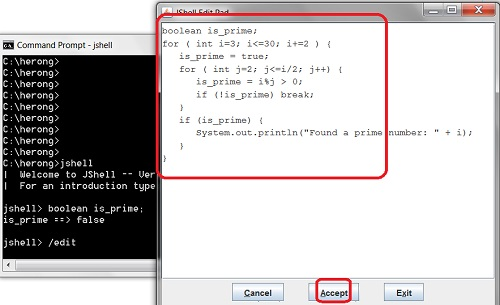"jshell> /edit" - Editing Code Snippet

This section provides a tutorial example on how to start the editor to enter and change a long code snippet in 'jshell'.

If you are tired of entering statements line by line at the command prompt, you use the "/edit" command to enter and modify the code snippet in a text editor:

```herong> jshell
|  Welcome to JShell -- Version 12.0.1
|  For an introduction type: /help intro

jshell> boolean is_prime;
is_prime ==> false

jshell>/edit

(enter the following code in the editor)
for ( int i=3; i<=30; i+=2 ) {
is_prime = true;
for ( int j=2; j<=i/2; j++) {
is_prime = i%j > 0;
if (!is_prime) break;
}
if (is_prime) {
System.out.println("Found a prime number: " + i);
}
}

(click "Accept" button on the editor)
Found a prime number: 3
Found a prime number: 5
Found a prime number: 7
Found a prime number: 11
Found a prime number: 13
Found a prime number: 17
Found a prime number: 19
Found a prime number: 23
Found a prime number: 29
```

Each time you click the "Accept" button, the code snippet will be executed one more time.

This is a nice tool, if you want to test a few lines of Java code. You can make a change, run it, change it again, and run it again quickly.jshell - Java Code Snippet Editor

Table of Contents